# Zeros on the $\sigma=1$ line

By the Euler Product, the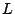-function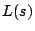does not vanish in the half-plane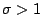. Thus, the simplest nontrivial assertion about the zeros ofis thatdoes not vanish on the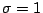line. Such a result is known as a Prime Number Theorem for. The name arises as follows. The classical Prime Number Theorem(PNT):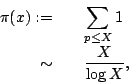where the sum is over the primes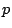, is equivalent to the assertion that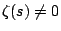when. The deduction of the PNT from the nonvanishing involves applying a Tauberian theorem to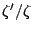. The Tauberian Theorem requires thatbe regular on, except for the pole at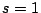.

The Prime Number Theorem for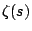was proven by Hadamard and de la Valee Poissin in 1896. Jacquet and Shalika [55 #5583] proved the corresponding result for-functions associated to automorphic representations on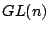. It would be significant to prove such a result for the Selberg Class.

Back to the main index for The Riemann Hypothesis.